# The Separation Principle in Linear Regression

Francisco J. Samaniego
University of California, Davis

Mitchell R. Watnik
University of Missouri-Rolla

Journal of Statistics Education v.5, n.3 (1997)

Copyright (c) 1997 by Francisco J. Samaniego and Mitchell R. Watnik, all rights reserved. This text may be freely shared among individuals, but it may not be republished in any medium without express written consent from the authors and advance notification of the editor.

Key Words: Aggregation; Baseball; Correlation; Independent variable; Projection.

## Abstract

In linear regression problems in which an independent variable is a total of two or more characteristics of interest, it may be possible to improve the fit of a regression equation substantially by regressing against one of two separate components of this sum rather than the sum itself. As motivation for this "separation principle," we provide necessary and sufficient conditions for an increased coefficient of determination. In teaching regression analysis, one might use an example such as the one contained herein, in which the number of wins of Major League Baseball teams is regressed against team payrolls, for the purpose of demonstrating that an investigator can often exploit intuition and/or subject-matter expertise to identify an efficacious separation.

# 1. Introduction

1 We will both motivate and illustrate the Separation Principle through the following real example. Suppose we wish to relate the number of wins, Y, achieved by a Major League Baseball team in a given season to the team's total payroll, X. Most baseball fans, and perhaps even many folks who barely recognize the game's existence, would readily believe that these two variables are positively related. If one fits a straight line to the (wins, payroll) data, one indeed finds that there is a significant positive relationship. One might be slightly disappointed to note that the strength of the relationship is not especially large (R2 is only around .3), but one may nonetheless assert that baseball owners do indeed buy wins; an extra million dollars spent on a team's payroll produces, on the average, about half a win over the course of a season. The analysis above might well be the endpoint of the study in question; indeed, reporting results at this stage is typical of many studies in which a variable Y is regressed, seemingly successfully, against a grand total X. The lesson we wish to drive home in this note is that one should not be so easily pleased.

2 By the Separation Principle, we mean the practice of recognizing and executing a beneficial separation of an "independent" variable X into two components, X1 and X2, one of which provides a better regression equation for Y than the variable X itself. Namely, if X is an aggregate, we should consider the components of X as possible regressors. Looking for situations in which R2 can be increased via separation is one way of finding candidates for improving a regression equation.

3 We believe that both the idea and the mechanics of separation should be taught in regression courses and should be borne in mind in regression applications involving aggregation. The discovery of a useful separation in such problems will, of course, typically rely on good intuition, and is, perhaps, more of an art than a science. The search for a good separation involves a subjective element -- that of identifying meaningful or interpretable components whose sum is X -- and a technical element -- that of verifying that one or the other of these components is a better regressor than X. This argues for the close collaboration of subject-matter and statistical researchers, an argument that is well supported by the application of the separation principle to our (wins, payroll) data. In our example, we concentrate on separating payroll into the payrolls for two distinct types of players: the pitchers, who are arguably the most important subset of a baseball team, and the non-pitchers, who are typically the offensive contributors to a team's success. As will be seen, it turns out that the payroll for pitchers is highly significant, while the other part of the payroll is not very helpful in predicting the number of wins.

# 2. Theoretical Results

4 Consider, now, the standard linear regression setting in which one is prepared to fit the model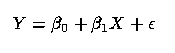(1)

to data. Suppose that the variable X can be written as a sum, that is, suppose that(2)

When will it be useful to fit the alternative models regressing Y against either X1 or X2? The following result provides a necessary and sufficient condition for an improved fit as measured by the coefficient of determination, R2.

5 Theorem: Assume that the vectors (Y, X1, X2) obey a standard linear regression model with uncorrelated errors, and let X = X1 + X2. Further, let R2(U,V) represent the coefficient of determination between the variables U and V, that is, let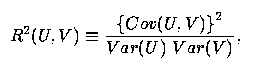(3)

where Cov(U,V) represents the covariance between U and V, etc. Then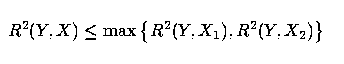(4)

if, and only if, the correlation between X1 and X2, Corr(X1, X2), satisfies the inequality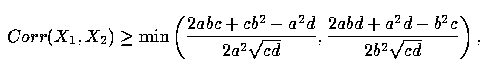(5)

where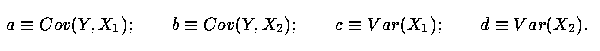(6)

The proof is given in the Appendix. We note that a similar result may be obtained using the sample estimates in place of the variance and covariance parameters. That is, substituting the statistics in place of the parameters would yield necessary and sufficient conditions for increasing the sample R2.

6 Before illustrating the Separation Principle in an example in which the theorem above applies, we pause briefly to discuss the appropriate interpretation of this result. First, it must be recognized that the theorem above is an existence theorem rather than a result that is useful in verifying that one has a good separation in hand. Rather than verifying that inequality (5) obtains, it will be easier in any real problem to see if a given separation is effective by running the two alternative regressions or by performing the multiple regression of Y on both X1 and X2 and testing the hypothesis=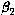. The real utility of this theorem is that it tells you what to look for; the theorem should be viewed as an exploratory tool rather than a model-fitting tool. In addition and as an added side-product, it may be the case that the multiple regression involving both X1 and X2 is substantially better than the individual simple regressions, but "substantially better" must also include consideration of parsimony and the significance of each variable. The theorem shows that a separation will produce at least a simple linear regression equation that is as good as, or better than, the original equation when the correlation between the separate components X1 and X2 is sufficiently high. Because of this, multicollinearity between X1 and X2 may be a concern in the multiple regression model. On the other hand, it is noteworthy that a positive correlation between them is not required -- the right hand side of (5) can be negative. Still, the theorem suggests that one might look for separations of X into a sum of positively correlated components.

7 In our baseball example, our intuition suggested that it would make sense to consider separating total payroll into pitchers' and hitters' payrolls. The fact that hitting and pitching payrolls tend to vary together as total payrolls vary across major league baseball suggests, via the theorem above, that this particular separation will be effective in producing a better regression equation. We will verify momentarily that this is indeed the case.

8 It is, of course, obvious that the regression of Y on the pair (X1, X2) must necessarily produce a higher R2 than the regression of Y on X; the latter regression is less general than the former, because it places an implicit restriction on the coefficients of X1 and X2. Most introductory regression texts treat the problem of comparing models of this type (see, for example, Neter, Kutner, Nachtsheim, and Wasserman 1996, p. 230). Structurally, the model in (1), with X = X1 + X2, resembles the standard "errors-in-variables" models discussed by, among others, Cochran (1968), Anderson (1984), Fuller (1987), and Whittemore (1989). The question of interest here, however, is whether or not one of these two variables, by itself, provides an improved regression equation. While a large correlation (in the sense of (5)) guarantees improvement, note that this improvement need not be strict, and that it is not monotonic in Corr(X1, X2). When that correlation is 1, for example, the regression of Y on X and that of Y on either Xi have identical coefficients of determination.

# 3. Geometry

9 Geometrically, the correlation between two vectors is equal to the cosine of the angle between them. Thus, if the vectors are close to being orthogonal, the correlation is low. Let Y* be the projection of the vector Y into the space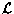generated by (X1, X2). The restriction we place on the model forces the angle between Y* and X to be a weighted average of the angles between Y* and X1 and Y* and X2, where the weights are the lengths of the two vectors X1 and X2. When the projection of the vector Y intodoes not lie between the vectors X1 and X2, removal of the restriction will give a better fitting regression line. When the projected Y, or its negative, does lie between X1 and X2, the geometric analog of equation (4) implies that we are better off using the total, X, only if the angle between X and Y* is smaller than the minimum of the angles between Y* and X1 and Y* and X2. Thus, if the angle between X and Y* is small, condition (5) will be hard to satisfy; that is, it will be hard to find X1 and X2 so that one of those two will be closer to Y* than X already is. For example, if X and Y* have correlation 1, only X1 and X2 having correlation 1 would satisfy condition (5). Geometrically, an analogous example is to have the projection of Y intobe a multiple of X. We would then need to have X1 = kX and X2 = (1 - k)X in order to satisfy condition (5).

10 Conversely, if X does not provide a good fit for Y, it may be to the investigator's advantage to separate X into X1 and X2. In that situation, it should be relatively easy to find a separation in which either X1 or X2 or possibly both give a better fit for Y than does X. A trivial example which demonstrates this point is the situation where X1 = Y + error and X2 = -Y + error. Then, the regression of Y on X will have a very low R2, while the regressions of Y on X1 and Y on X2 will tend to have high R2.

# 4. The Baseball Example

11 Let us now examine the question of how a baseball team's performance, that is, the number of wins in a season, is related to the team's payroll. As we have mentioned, the first (and perhaps last) pass at this problem might regress wins against total payroll. The data on the wins and payroll, in millions of dollars, of each of the twenty-eight Major League Baseball teams that played in the 1995 season are shown in Table 1. Also displayed in the table is the separation of interest, that is, the payroll for pitchers and for hitters on each of these teams. The variable we have labeled as "total payroll" represents the total team payroll as of August 31, 1995, and is taken from the November 17, 1995, issue of USA Today.

Table 1. Performance/Salary Data for Major League Baseball teams in 1995. (Salaries are in millions of dollars.)

```                                    Total   Pitchers'  Hitters'
Team                   Wins  Payroll   Payroll    Payroll
Boston Red Sox          86     38.0      16.8      21.2
New York Yankees        79     58.1      29.5      28.6
Baltimore Orioles       71     48.9      18.6      30.3
Detroit Tigers          60     28.7       5.7      23.0
Toronto Blue Jays       56     42.1      12.3      29.8
Cleveland Indians       100    39.9      16.8      23.1
Kansas City Royals      70     31.2      15.0      16.2
Chicago White Sox       68     40.7      10.0      30.7
Milwaukee Brewers       65     16.9       6.5      10.4
Minnesota Twins         56     15.4       1.3      14.1
Seattle Mariners        78     37.9      16.4      21.5
California Angels       78     33.9      17.3      16.6
Texas Rangers           74     35.7      12.5      23.2
Oakland Athletics       67     33.4       7.5      25.9
Atlanta Braves          90     47.3      23.3      24.0
Philadelphia Phillies   69     30.3       7.4      22.9
New York Mets           69     13.1       7.3       5.9
Florida Marlins         67     22.8      11.6      11.2
Montreal Expos          66     13.1       5.6       7.5
Cincinnati Reds         85     47.5      24.2      23.3
Houston Astros          76     33.5      15.8      17.7
Chicago Cubs            73     36.4      10.7      25.7
St.  Louis Cardinals    62     28.4      10.8      17.6
Pittsburgh Pirates      58     17.7       4.1      13.6
Los Angeles Dodgers     78     36.7      18.7      18.0
Colorado Rockies        77     38.1      16.8      21.3
San Diego Padres        70     24.9       3.4      21.5
San Francisco Giants    67     33.7       7.4      26.3
```

12 Letting Y = regular season wins in 1995, X = total payroll, X1 = pitchers' payroll, and X2 = hitters' payroll = X - X1, the following regression equations were obtained: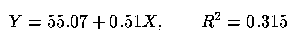(7)

and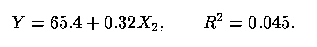(9)

In this example, the constants a, b, c, and d in our theorem take the sample values 50.13, 14.48, 46.47, and 44.79, respectively. The correlation between X1 and X2 is about 0.38. It is easy to verify that the correlation between pitchers' and hitters' payroll satisfies inequality (5) as it must, of course, because the coefficients of determination above clearly satisfy inequality (4).

13 In this example, one might be satisfied with the finding that the total number of wins is reasonably well explained as a function of total payroll. From that, we might give the run-of-the-mill advice to team owners to spend if they want to win. It is possible, however, to give owners a better piece of advice -- spend wisely, invest in good pitching. It bears keeping in mind, of course, that in applications such as the one under consideration, the best fitting regression equation may not be as useful in practice as a suboptimal one based on variables that are easier to control. In the present example, owners might find that a high-priced pitcher will refuse to sign with a team whose hitting payroll is too small (we are indebted to a referee for this point). In this example, however, the correlation between X1 and X2 is low enough to make us believe that owners could spend more on pitching without necessarily increasing the amount paid to hitters.

14 Assuming, however, that an owner can sign any player given enough money, one can imagine that the same separation approach could also be used to separate the hitting payroll into more descriptive subgroups such as "leadoff hitter and clean-up hitter" and "other" to get a much better fit on how hitting payroll relates to wins. So, for example, if the former category has strong positive correlation with number of wins while the latter showed negative correlation, the owner could invest money in these key players and not worry about the others. Similarly, it is also possible that pitching payroll could be further separated into more descriptive subgroups, one of which might provide an even better fit than the regression line in (8) above.

# 5. Other Examples

## 5.1. Relative Income

15 As another example, we consider the relative income hypothesis of Duesenberry (1949). We know that the aggregate consumption at time t, Ct, in an economy is autoregressive and also depends upon consumer income, Yt. One might be interested in estimating how much of an effect consumer income has on consumption after eliminating the autoregressive effect. We thus define Ct* as the residuals from the model of Ct regressed on Ct-1.

16 In the relative income hypothesis, however, an economist separates income into two parts: highest level of income achieved prior to the current year, Z1t, and the difference between the current year's income and the previous highest level of income, Z2t = Yt - Z1t (Doran 1989, p. 253). The latter part of the separation might be viewed as discretionary income and, therefore, its coefficient would measure consumers' short run propensity to consume. Doran (1989, p. 244) provides data for Australian consumption and expenditures for the fiscal years 1949-1980.

17 We obtained the following regression equations: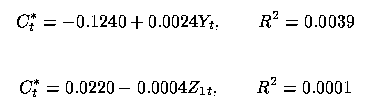and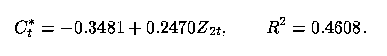Here, then, the separation process succeeds in identifying a regressor variable that is more highly correlated with the response. This is not surprising, though, because the total income has such a low correlation with the response. In addition, this separation makes intuitive sense since the response is mostly change in consumption, while Z2t is a proxy for change in income.

## 5.2. Interest and Investment

18 We now consider modelling investment, Y, on Gross National Product (GNP) and interest rate, I. Greene (1993, p. 174) provides data for the years 1968-1982 and recommends the inclusion of a time trend, T = 1, ..., 15, indicative of the year of the study; i.e., T = year - 1967. One might separate the interest rate into two parts: inflation rate, F, and interest above inflation, I* = I - F (cf. Greene 1993, p. 187).

19 We obtained the following regression equations:and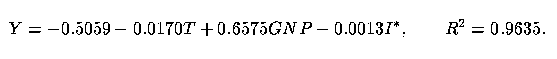Here, then, the separation is not beneficial. It can be seen that the estimates of the coefficients associated with F and I* are equal. Although I is a significant regressor (t = -2.29), neither subcomponent is significant. The R2 for the regression of Y on just T and GNP is 0.9593.

## 5.3. Fuel Consumption

20 Consider modelling the log of fuel consumption by state, Y, as a linear function of the log of the population of that state, X1, the tax rate on fuel in cents per gallon, X2, the per capita income in that state, X3, and the amount of federally-funded roadway in that state in thousands of miles, X4. These data come from Weisberg (1985, pp. 35-36). The log of the response was taken so that the variance of the residuals would not depend upon the independent variables. One might separate the log of the total population, X1, into the log of the population with drivers licenses, Z1, and Z2 = X1 - Z1, which is the negative of the log of the proportion of the population with drivers licenses.

21 We obtained the following regression equations: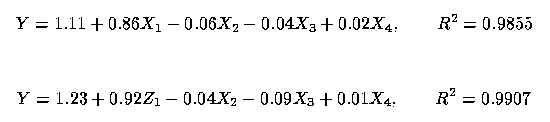and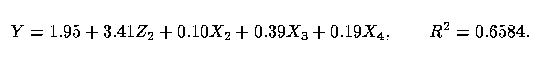Here, the separation process identifies a better regressor than just the total. Clearly, in this model the log of the population with drivers licenses is a better regressor than simply the log of the population.

# 6. Concluding Remarks

22 The idea discussed here, namely that one should consider components that make up an aggregate as possible regressor variables, can be presented with profit in introductory regression classes, particularly as part of discussions of model building strategies. Indeed, it may be offered in the context of stimulating real-life examples that draw from sports, business, politics, and the like. This idea can also prove useful in regression problems arising in statistical consulting and collaborative work. Regression is, after all, a methodology for finding the best fitting model from a possibly large class of models. We have seen here that, when that class of models includes a variable X that is itself a grand total or sum, the class of models that we should consider is larger than the traditional one (i.e., all subsets of a fixed set of k regressors). Separating X, where possible, may well contribute to the development of a better model.

23 The success of the strategy of separating a variable X into components X1 and X2 will of course depend on the extent to which one is free to disaggregate the raw data that resulted in the total X. To take maximal advantage of the separation principle, one would like to be dealing with raw data on a set of individual units that can be partitioned into two separate groups quite freely. It is clear that the opportunity exists for mining the data to obtain separations in which one component Xi is highly correlated with Y. While this might be productive as an exploratory technique, it will only be useful when that separation corresponds to a reasonable, interpretable partition of the data. The best separations, like any other set of independent regressors, should come from knowledge of the problem rather than from simply massaging the data. Also, students will appreciate that their knowledge of the non-statistical problem can be of great assistance in their model building. As always, care must be taken to avoid overfitting the data. When separation is used as an exploratory device, it is wise to seek to validate any relationship discovered thereby with a second, independent dataset. Additionally, it may be interesting to study the behavior of the separation principle using other measures for goodness of fit. We hope to do this in a future investigation.

24 The separation principle highlights the possibility of better explaining the variability of the dependent variable in a linear regression model by seeking a suitable disaggregation of the independent variable. While we have emphasized the practice of checking whether R2 is greater in the separated regression than in the aggregated regression, it should be clear that, even when it results in an apparently useful bifurcation, the separation principle does not, by itself, represent a comprehensive statistical modeling strategy. We advocate the use of the coefficient of determination as a tool in searching for potentially useful separations, but we recommend that any candidate separation be scrutinized using the standard battery of model building tools and diagnostics. It is necessary, as always, to pay close attention to the ŕ priori appropriateness of the regression specification adopted, the properties of the disturbance term, and the statistical significance of regression estimates. In a multiple regression setting, one would also wish to determine whether the increase in R2 is itself statistically significant. In using the separation principle as a teaching device, it is important to draw students' attention not only to what it does but also to what it does not do.

## Acknowledgments

The authors would like to thank Alan Fenech, three anonymous referees, and the editor for their helpful suggestions.

# Appendix

Proof of the Theorem: We wish to establish a necessary and sufficient condition on Cov(X1, X2) for the following inequality to obtain: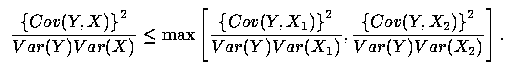(A1)

First, consider the left-hand side of inequality (A1). Using the notation from the equations in (6), and letting e = Cov(X1, X2), we have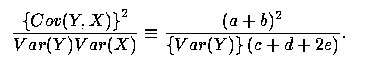(A2)

We thus need to show that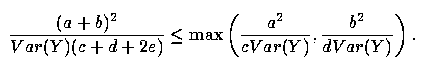(A3)

Because both sides of (A3) are necessarily positive, that inequality is equivalent to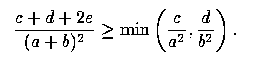(A4)

But (A4) holds if, and only if,(A5)

a statement which is equivalent to (5).

# References

Anderson, T. W. (1984), "Estimating Linear Statistical Relationships," Annals of Statistics, 12, 1-45.

Cochran, W. G. (1968), "Errors of Measurement in Statistics," Technometrics, 10, 55-83.

Doran, H. E. (1989), Applied Regression Analysis in Econometrics, New York: Marcel Dekker, Inc.

Duesenberry, J. S. (1949), Income Saving and the Theory of Consumer Behavior, Cambridge, MA: Harvard University Press.

Fuller, W. A. (1987), Measurement Error Models, New York: John Wiley.

Greene, W. H. (1993), Econometric Analysis, New York: Macmillan Publishing Co.

Neter, J., M. H. Kutner, C. J. Nachtsheim, and W. Wasserman (1996), Applied Linear Regression Models (3rd ed.), Chicago, IL: Richard D. Irwin, Inc.

Weisberg, S. (1985), Applied Linear Regression, New York: John Wiley and Sons.

Whittemore, A. S. (1989), "Errors-in-Variables Regression Using Stein Estimates," The American Statistician, 43, 226-228.

Francisco J. Samaniego
Division of Statistics
University of California, Davis
Davis, CA 95616

fjsamaniego@ucdavis.edu

Mitchell Watnik
Department of Mathematics and Statistics
University of Missouri-Rolla
Rolla, MO 65409

mwatnik@umr.edu